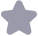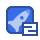### Introduction to Algorithms (算法导论 潘金贵译）第三版答案收藏

Introduction to Algorithms (算法导论 潘金贵译）第三版答案
o(nn) Alp. qI Alq +1.r R x R A[i R L R R R R L R 又=L[y=R L[i],L+1],L[+2],,L +1 n1-i+1 (A p,r) q=L(P+r)/2 (A, p, g) (A,q+1,r) (A, p,, r) (A, P, g,r) n1=g-p+ L[1.n1+1]R[1..n2+1 L[i]= alp ti-1 RLi= Alg+jl R[n2+1]=∞ 0 k=p r Rll<Lil +n1-i+1 L[≤R[j Ak= l[ i=i+1 Ak= rli J=J (A,1,n) R[门] R[门] R[门] R R o(nn) (n +a C1.C2,H0 a 1 n1+a> n22|a 0≤-≤n+a≤2nt b>0 ≤(n+a)≤(2m)b b +a)<2 T(m)T(m)≥O(n2) 7(m)≥f(n) g(m)=0 O( 2 O(2) 0 0<2 n+1 22n≠O(2 C70> 0 0< 12>n 2 1 (f(m)=O(n) ck no n≥n0f(m)≤cm (f(n))≤k (f(n))=O(n) (f(m))=0(n)f (n!)=⊙(nn n1<n+1≤2n >2 (「n1!)≠O(m) AC a,b>0 n2=0(1 n n 2 b 1 n)=0 n (n1!)=O(m) 「n1! kT(n/3)+Q(n2) k k=2 T(n)=o( T(m)=0( k<2 k<9 T(m)=0(n7) 3 k>2 T(m)=⊙(n k>9 T(n=o( k k<37≈21.85 k21 k=21 Q(n3k)=((n321)=O(20 21≈2.77 n→(1/3) (1/3)2 (1/3)n=1k cn cn 3n=&(nn T(n)=T(an)+T((1-a)n)+cn )=7(n/3)+T(2n/3)+ 0<1-a≤1/21/2≤a<1 Con cn 1/(1-a) coin ca(1-a)n ca(1-a)n c(1-a)2n Gummi. cn O(nn) Cn (n1/(1-a)n)=!2(nn) cn O(n /a n)=o(nn T(n)=o(nn) T(n)≤dnn T(n)= T(an)+T((1-a)n)+cn k dan (an)+d(1-aon((1-a)n)+cn dan a+dan n+d(i-an (1-a)+d(I-a)nn+cn +dn(ac+(1-a)(1-a)+cn dn(c+(1-a)(1-c)+cn≤0 d(ac+(1-a)(1-a) 1/2≤<10<1-c≤1/2 c+(1-)(1-c)<0 C c+(1-)(1-c) +-(1-a)(1 T(n)> dn n 0<d T (1,2,,n) 兀 n 1.2 X=X1+X +X 1/n x;=1}=1/n =1/n

...展开详情

一个资源只可评论一次，评论内容不能少于5个字
2014-09-27
回复
2014-04-12
回复fate_wheel 答案很详细， 就是不够全面 后面就没了 不过参考价值还是很高的
2013-12-27
回复
2013-12-19
回复
2013-07-16
回复
2013-04-08
回复
地狱烈火关注 私信 TA的资源
上传资源赚积分,得勋章
最新推荐Introduction to Algorithms (算法导论 潘金贵译）第三版答案 50积分/C币 立即下载
1/70试读已结束，剩余50页未读...

50积分/C币 立即下载 ＞